Test: Second Law of Thermodynamics - 3

# Test: Second Law of Thermodynamics - 3

Test Description

## 10 Questions MCQ Test Thermodynamics | Test: Second Law of Thermodynamics - 3

Test: Second Law of Thermodynamics - 3 for Mechanical Engineering 2023 is part of Thermodynamics preparation. The Test: Second Law of Thermodynamics - 3 questions and answers have been prepared according to the Mechanical Engineering exam syllabus.The Test: Second Law of Thermodynamics - 3 MCQs are made for Mechanical Engineering 2023 Exam. Find important definitions, questions, notes, meanings, examples, exercises, MCQs and online tests for Test: Second Law of Thermodynamics - 3 below.
Solutions of Test: Second Law of Thermodynamics - 3 questions in English are available as part of our Thermodynamics for Mechanical Engineering & Test: Second Law of Thermodynamics - 3 solutions in Hindi for Thermodynamics course. Download more important topics, notes, lectures and mock test series for Mechanical Engineering Exam by signing up for free. Attempt Test: Second Law of Thermodynamics - 3 | 10 questions in 30 minutes | Mock test for Mechanical Engineering preparation | Free important questions MCQ to study Thermodynamics for Mechanical Engineering Exam | Download free PDF with solutions
 1 Crore+ students have signed up on EduRev. Have you?
Test: Second Law of Thermodynamics - 3 - Question 1

### The Carnot cycle is unpredictable because it

Test: Second Law of Thermodynamics - 3 - Question 2

### An engine operates between temperature limits of 900 K and T2 and another between T2 and 400 K for both to be equally efficient T2 will be equal to

Detailed Solution for Test: Second Law of Thermodynamics - 3 - Question 2

For efficiency to be equal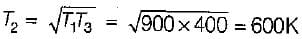Test: Second Law of Thermodynamics - 3 - Question 3

### An inventor claims that a heat engine has the following specifications: Developed = 50 kW Fuel burned per hour = 3 kg Heating value of fuel = 7500 kJ/kg Temperature limit = 627°C and 27°C Cost of fuel = Rs. 30/kg Power the performance of his engine is

Detailed Solution for Test: Second Law of Thermodynamics - 3 - Question 3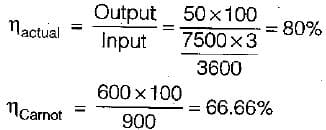Since actual efficiency of the engine is greater than Carnot hence engine is not possible.

Test: Second Law of Thermodynamics - 3 - Question 4

Three engines A, B and C operating on Carnot cycle respectively use air, steam and Helium as the working fluid. If all the engine operates within the same temperature limit, then which engine will have highest efficiency.

Detailed Solution for Test: Second Law of Thermodynamics - 3 - Question 4

Since efficiency of the engine purely depends upon source and sink temperatures and independent of the working substances. All the engines have same efficiency.

Test: Second Law of Thermodynamics - 3 - Question 5

If a heat engine gives an output of 3 kW.when the input is 10000J/sec then the thermal efficiency of the engine will be

Detailed Solution for Test: Second Law of Thermodynamics - 3 - Question 5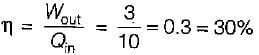Test: Second Law of Thermodynamics - 3 - Question 6

A Carnot engine operates between 27°C and 327°C. If the engine produces 300 kJ of work, what is the entropy change during heat rejection?

Detailed Solution for Test: Second Law of Thermodynamics - 3 - Question 6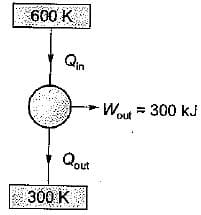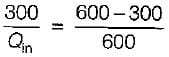Oin = 600 KJ
Qout = 300K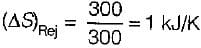Test: Second Law of Thermodynamics - 3 - Question 7

The following heat engine produces power of 100,000 kW. The heat engine operates between 800 K and 300 K. It has a thermal efficiency equal to 50% of that of the Carnot engine for the same temperatures. The rate at which heat is absorbed from the hot reservoir is

Detailed Solution for Test: Second Law of Thermodynamics - 3 - Question 7

Power produced by engine = Workdone(W) = 100,000 k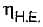= 50% of Carnot cycle or engine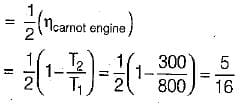Also,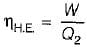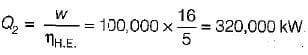= 320 MW

Test: Second Law of Thermodynamics - 3 - Question 8

A heat engine operates at 75% of the maximum possible efficiency. The ratio of the heat source temperature (in K) to the heat sink temperature (in K) is 5/3. The fraction of the heat supplied that is converted to work is

Detailed Solution for Test: Second Law of Thermodynamics - 3 - Question 8

Given,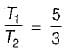Efficiency,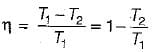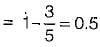Also η = Work done/Heat supplied
Since the engines uses only 75% of the heat made available to it, therefore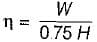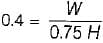W = 0.3 x H

Test: Second Law of Thermodynamics - 3 - Question 9

Which of the following represents the Carnot cycle (ideal engine)?

Detailed Solution for Test: Second Law of Thermodynamics - 3 - Question 9

Carnot cycle consist of two Isentropics and two isothermals.

Test: Second Law of Thermodynamics - 3 - Question 10

A reversible power cycle is used to drive a reversible heat pump cycle. The power cycle takes in Q1 heat units at T1 and reflects Q2 at T2. The heat pump abstracts Q4 from sink at T4 and discharges Q3 at T3. The ratio of Q4/Q1 (in terms of four temperature) is

Detailed Solution for Test: Second Law of Thermodynamics - 3 - Question 10

The power cycle operating between temperature T1, and T2 and heat pump is operating between temperature T3 and T4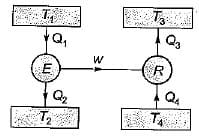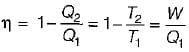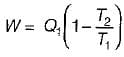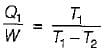COP of reversible pump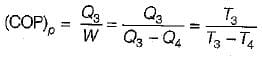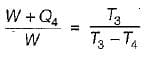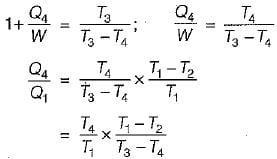## Thermodynamics

33 videos|54 docs|36 tests
 Use Code STAYHOME200 and get INR 200 additional OFF Use Coupon Code
Information about Test: Second Law of Thermodynamics - 3 Page
In this test you can find the Exam questions for Test: Second Law of Thermodynamics - 3 solved & explained in the simplest way possible. Besides giving Questions and answers for Test: Second Law of Thermodynamics - 3, EduRev gives you an ample number of Online tests for practice

## Thermodynamics

33 videos|54 docs|36 tests